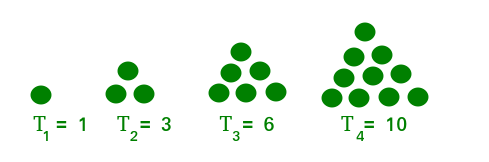# Find the sequence number of a triangular number

Given an integer N print the sequence number of the given Triangular Number. If the number is not a triangular number then print -1.

A number is termed as a triangular number if we can represent it in the form of a triangular grid of points such that the points form an equilateral triangle and each row contains as many points as the row number, i.e., the first row has one point, the second row has two points, the third row has three points and so on.
First 10 tringular number are: 1, 3, 6, 10, 15, 21, 28, 36, 45, 55.Examples:

Input: N = 21
Output:6
Explanation:
Since 15 is a 6th Tringular Number.

Input: N = 12
Output:-1
Explanation:
Since 12 is not a tringular Number

## Recommended: Please try your approach on {IDE} first, before moving on to the solution.

Approach:

1. Since tringular numbers are sum of natural numbers so can be generalise as quadratic equation.
2. (X*(X+1))/2 = N
X = (-1 + (1 + 8*N) ) /2
X = -0.5 + (0.25 + 2*N)^1/2

## C++

 `// C++ code to print sequence  ` `// number of a triangular number ` `#include ` `using` `namespace` `std; ` ` `  `int` `main() ` `{ ` `    ``int` `N = 21; ` `    ``int` `A = ``sqrt``(2 * N + 0.25) - 0.5; ` `    ``int` `B = A; ` ` `  `    ``// If N is not tringular number ` `    ``if` `(B != A) ` `        ``cout << ``"-1"``; ` `    ``else` `        ``cout << B; ` `} ` ` `  `// This code is contributed by yatinagg `

## Java

 `// Java code to print sequence  ` `// number of a triangular number ` `import` `java.util.*; ` `class` `GFG{ ` `     `  `public` `static` `void` `main(String args[]) ` `{ ` `    ``int` `N = ``21``; ` `    ``int` `A = (``int``)(Math.sqrt(``2` `* N + ``0.25``) - ``0.5``); ` `    ``int` `B = A; ` ` `  `    ``// If N is not tringular number ` `    ``if` `(B != A) ` `        ``System.out.print(``"-1"``); ` `    ``else` `        ``System.out.print(B); ` `} ` `} ` ` `  `// This code is contributed by Akanksha_Rai `

## Python3

 `# Python3 code to print sequence  ` `# number of a triangular number ` ` `  `import` `math ` ` `  `N ``=` `21` `A ``=` `math.sqrt(``2` `*` `N ``+` `0.25``)``-``0.5` `B ``=` `int``(A) ` ` `  `# if N is not tringular number ` `if` `B !``=` `A: ` `    ``print``(``-``1``) ` `else``: ` `    ``print``(B)          `

## C#

 `// C# code to print sequence  ` `// number of a triangular number ` `using` `System; ` `class` `GFG{ ` `     `  `public` `static` `void` `Main() ` `{ ` `    ``int` `N = 21; ` `    ``int` `A = (``int``)(Math.Sqrt(2 * N + 0.25) - 0.5); ` `    ``int` `B = A; ` ` `  `    ``// If N is not tringular number ` `    ``if` `(B != A) ` `        ``Console.Write(``"-1"``); ` `    ``else` `        ``Console.Write(B); ` `} ` `} ` ` `  `// This code is contributed by Code_Mech `

Output:

```6
```

Time Complexity: O(1)
Auxillary Space: O(1)My Personal Notes arrow_drop_upCheck out this Author's contributed articles.

If you like GeeksforGeeks and would like to contribute, you can also write an article using contribute.geeksforgeeks.org or mail your article to contribute@geeksforgeeks.org. See your article appearing on the GeeksforGeeks main page and help other Geeks.

Please Improve this article if you find anything incorrect by clicking on the "Improve Article" button below.

Practice Tags :

Be the First to upvote.

Please write to us at contribute@geeksforgeeks.org to report any issue with the above content.